# 因式分解二次式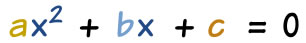(abc可以是任何值，但 a 不能是零。）

"因式分解" （也叫作"分解因式"）一个二次式是去：

## 例子

x2 + 3x − 4 的因式是：

(x+4)(x−1)

 (x+4)(x−1) = x(x−1) + 4(x−1) = x2 − x + 4x − 4 = x2 + 3x − 4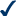(x+4)(x−1) 相乘是叫展开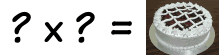这有点像找出什么材料使一个蛋糕好吃。 有时这绝对不明显！

## 公因式

### 例子：6x2 − 2x = 0 的因式是什么？

262 的公因式：

2(3x2 − x) = 0

xx2x 的公因式：

2x(3x − 1) = 0

• x = 0 时，2x = 0
• x = 1/3 时，3x − 1 = 0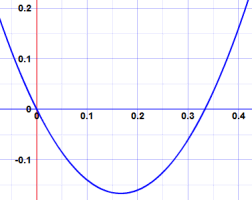## 猜。。。。。。测

### 例子：2x2 + 7x + 3 的因式是什么？

(2x+3)(x+1) = 2x2 + 2x + 3x + 3
= 2x2 + 5x + 3
（错）

(2x+7)(x−1) = 2x2 − 2x + 7x − 7
= 2x2 + 5x − 7
（还是不行）

(2x+9)(x−1) = 2x2 − 2x + 9x − 9
= 2x2 + 7x − 9
（拿石头砸自己的脚）

## 简单情况下可用的方法、找两个积是等于 give ac 的数（积是 a 乘 c），同时这两个数的和是 b

ac 是 2×3 = 6，b 是 7

6 的因数有 1、2、3 和 6。

、以这对因数重写二次式的中间部分：

2x2 + 6x + x + 3

、把前两项与后两项分开来分解：

2x(x+3) + (x+3)

、若我们没做错，应该有一个很明显的公因式。

 开始 2x(x+3) + (x+3) 等于： 2x(x+3) + 1(x+3) 故此： (2x+1)(x+3)

 2x2 + 7x + 3 2x2 + 6x + x + 3 2x(x+3) + (x+3) 2x(x+3) + 1(x+3) (2x+1)(x+3)

### 例子： 6x2 + 5x − 6

、ac 是 6×(−6) = −36，b 是 5

−4×9 = −36 和 −4+9 = 5

、重写 5x，用 −4x 和 9x：

6x2 − 4x + 9x − 6

、分解头两项和后两项：

2x(3x − 2) + 3(3x − 2)

、公因式是 (3x − 2)：:

(2x+3)(3x − 2)

### 例子：ac = −120 和 b = 7

120 的因数是 （正与负）：

1、2、3、4、5、6、8、10、12、15、20、24、30、40、60 和 120

• −10 x 12 = −120，但是 −10+12 = 2 （错）
• −8 x 15 = −120，并且 −8+15 = 7 （行了！）

## 为什么要分解因式？

### 例子： 6x2 + 5x − 6 的根（零）是什么？

(2x + 3)(3x − 2)

x = −3/2 时，(2x + 3) 亦等于零，

x = 2/3 时，(3x − 2) 亦等于零

−3/22/3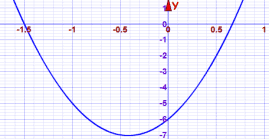## 画图

### 例子：（续）−3/22/3

## 通用解法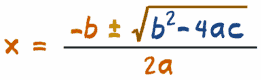a(x − x+)(x − x)

### 例子： 6x2 + 5x − 6 的根是什么？

x = (−b ± √[b2 − 4ac]) / 2a

x = (−5 ± √[52 − 4×6×(−6)]) / 2×6

= (−5 ± √[25 + 144]) / 12

= (−5 ± √169) / 12

= (−5 ± 13) / 12

x+ = (-5 + 13) / 12 = 8/12 = 2/3,

x = (-5 13) / 12 = −18/12 = −3/2

（和我们上面得到的答案是相同的）

6(x − 2/3)(x + 3/2)

3(x − 2/3) × 2(x + 3/2) = (3x − 2)(2x + 3)

（谢谢 "mathsyperson" 提供部分内容）